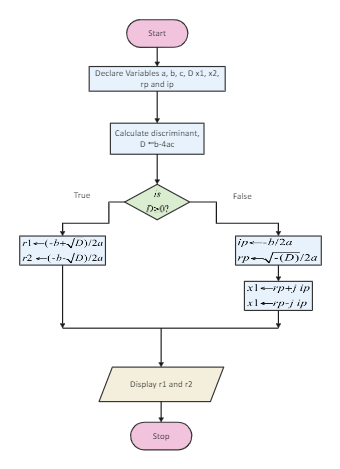Template Community / Declare Variables Algorithm Flowchart

# Declare Variables Algorithm Flowchart

Share
Duplicate2
0
Report
Publish time：07-16-2021
In Mathematics, the discriminant is a parameter of an object or System calculated as an aid to its classification or solution. In the following case of a quadratic equation ax^2 + bx + c = 0, the discriminant is b^2 - 4ac; for a cubic equation x^3 + ax^2 + bx + c = 0, the discriminant is a^b^2 + 18abc - 4b^3 - 4a^3c - 27c^2. As suggested in the algorithm diagram below, the discriminant can be positive, zero, or negative, and this determines how many solutions there are to be given a quadratic equation. It should be noted here that a positive discriminant indicates that the quadratic has two distinct real numbers. A discriminant of zero indicates that the quadratic has a repeated real number solution. Furthermore, a negative discriminant indicates that neither of the solutions is real numbers.
CreatorCharlottePost
Recommended TemplatesPublish
Your
Diagram